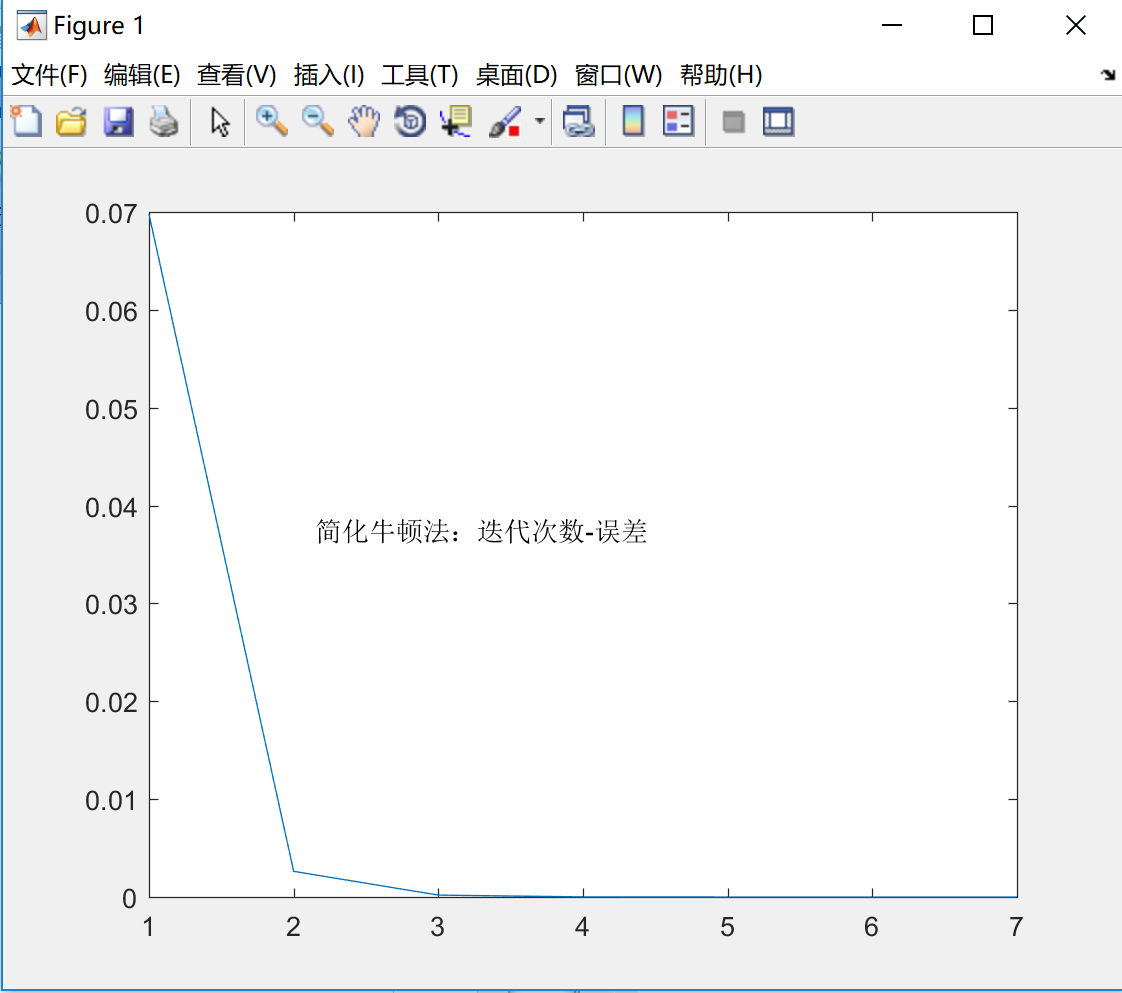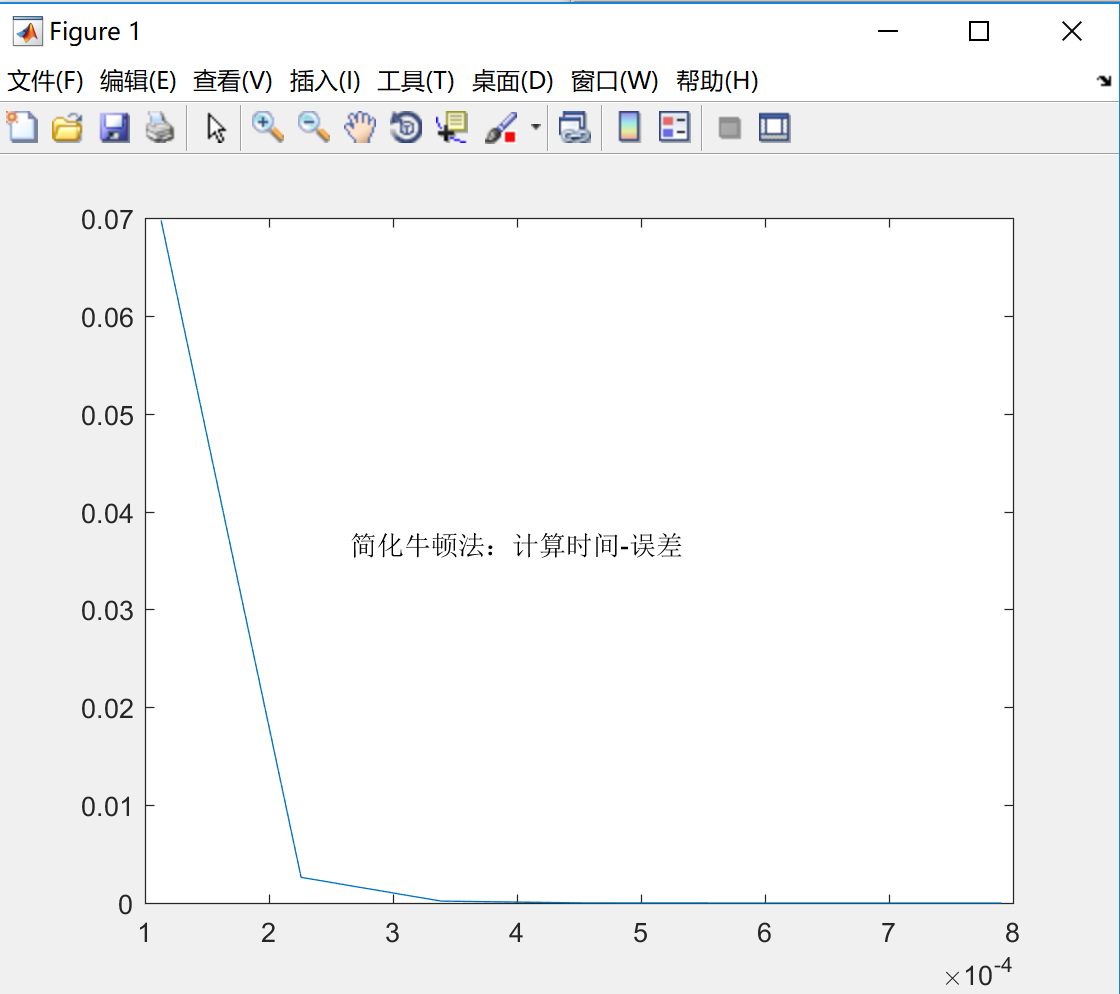# Halo

A magic place for coding

0%

## 介绍

在前面我们提到了牛顿法求解非线性方程，但是牛顿法有一个明显的缺点，就是在每一步迭代中都要计算函数值和一阶导数，这个计算量是巨大而且困难的，因此我们希望减少这个计算，对牛顿法进行了改进–简化牛顿法

## 分析

简化牛顿法是牛顿法的变种，原因在于，牛顿法中每一步都需要计算$f(x_k)$和$f^{‘}(x_k)$，这是需要很大计算量的。除此之外，牛顿法中的初始近似$x_0$只在精确解$x^*$附近才能保证收敛。简化牛顿法就是为了解决这个问题的。首先给出简化牛顿法的迭代公式：
$$x_{k+1} = x_k - Cf(x_k),$$

若$|\phi^{‘}(x)| = |1-Cf^{‘}(x)| < 1$，即取$0 < Cf^{‘}(x) < 2$在根附近成立，则该迭代法局部收敛。
同时取$C = \frac{1}{f^{‘}(x_0)}$，这样就只需要在第一步计算$f^{‘}(x_0)$，大大减少了计算量。其几何意义是用斜率为$f^{‘}(x_0)$的平行弦与$x$轴交点作为$x^*$的近似解。

## 代码实现

Matlab代码：

其中myFun是需要求解的非线性方程。

## 小结

对上面编写的代码进行了简单的数值实验，结果如下：简化牛顿法比牛顿法更有应用价值，对简化牛顿法的分析和讲解就到这里了，谢谢！

#### 参考资料：

1.数值分析（第5版） 李庆扬，王能超，易大义

Welcome to my other publishing channels